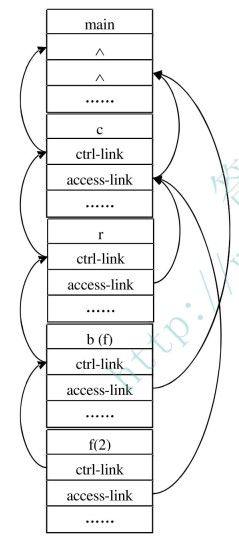## 运行时内存的划分

1）过程调用的序列和活动树的前序遍历相对应；

2）过程返回的序列和活动树的后序遍历相对应；

3）亲节点是未结束活动，且路径顺序即调用顺序

# 名称 作用
1 临时数据 存放表达式中间结果
2 局部数据 存放局部数据。详见下文。
3 机器状态 调用前的状态，如之前的寄存器等，以便恢复现场
4 访问链 指向外层的作用域栈帧。由于只和代码有关，又叫静态链
5 控制链 指向调用方的（上一个）栈帧（比如 RSP，存放上个帧栈顶）由于在运行过程中不断压栈时形成，又叫动态链
6 实参 用于存放主调函数为被调用函数所提供的实参信息
7 返回值 被调用函数用来为主调函数存放返回值的域## 变量的存储和分配

### 不可嵌套声明语言

（1）全局变量放在静态区。访问时使用固定地址。 （2）其它变量放在栈顶。一定是栈顶活动的局部变量。

### 可嵌套声明语言

function p(params) {
let lp = 0;
function q() {
return lp + 1;
}
lp = q();
}


## 传参机制

• a [i] a 代表存储单元的地址，即左值
• a [j] b 代表存储单元的内容，即右值

### 传值调用

int max(int x, int y) {
bool ret = x > y ? x : y;
x = 10; // 这一句不会产生任何效果
return ret;
}


### 引用调用

void swap(int &x, int &y)
{
int temp;
temp = x; /* 保存地址 x 的值 */
x = y;    /* 把 y 赋值给 x */
y = temp; /* 把 x 赋值给 y  */

return;
}


C++ 支持引用调用，C 不支持。

1. 如果实参是具有左值的名字或表达式， 则传递这个左值本身。
2. 如果实参是没有左值的表达式（如 a+b 或 2 等），则分配临时空间，计算完成后传递此临时空间地址

### 复制恢复

int y;
calling_procedure()
{
y = 10;          // 1
copy_restore (y); // 2 传递 y 的值
printf y;        // 7 prints 99
}
copy_restore (int x) // 3 x 是从外面复制进来的
{
x = 99; // 4 外面的 y 依旧是 10
y = 0;  // 5 外面的 y 变成 0
// 6 把 x 的值复制出去，变成外面的 y 的值
}

program copy_ref(input, output);
var a, b: integer;
procedure exchange(var x, y: integer);
var temp: integer;
begin
temp:=x;
x:=y;
y:=temp
end;
begin
a:=1; b:=2;
exchange(a,b);
writeln('a=',a); writeln('b=',b)
end


a = 2
b = 1


• 过程调用时，调用过程对实参求值，将实参的右值传递给 被调用过程，写入其活动记录的参数域中 (copy in)， 并记 录与形参相应的实参的左值。
• 被调用过程执行时，对形参的操作在自己的活动记录的参 数域空间上进行。
• 控制返回时，被调用过程根据所记录的实参的左值把形参 的当前右值复制到相应实参的存储空间中 (copy out) 。 当 然，只有具有左值的那些实参的值被复制出来。

### 传名调用

object Test {
def main(args: Array[String]) {
delayed(time());
}

def time() = {
println ("获取时间，单位为纳秒")
System.nanoTime
}
def delayed( t: => Long ) = {
println ("在 delayed 方法内")
println ("参数：" + t)
t
}
}


   def main(args: Array[String]) {
println ("在 delayed 方法内")
println ("参数：" + time ())
time()
}

#define MAX(X,Y) X>Y?X:Y

main(){
int a = 1>2?1:2
int b = MAX(2,3)
}


\$scala Test